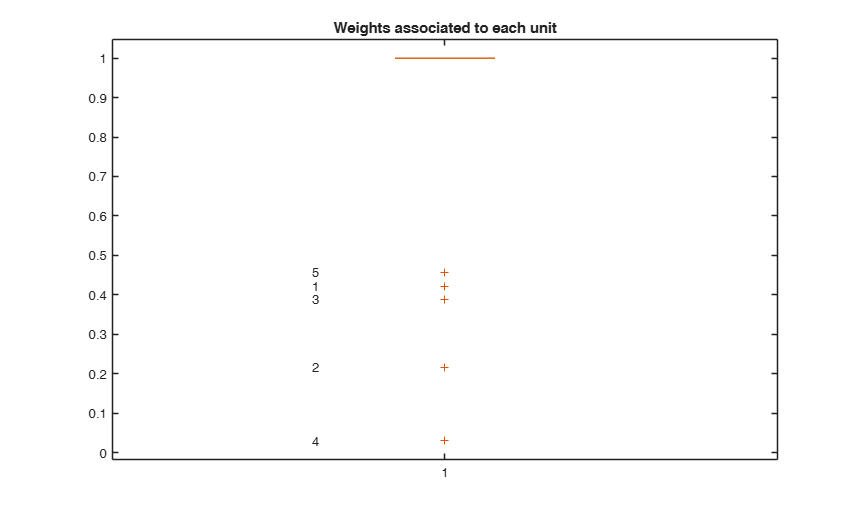VIOM

VIOM computes weights estimates under Variance-Inflation Model

Description

out =VIOM(y, X, dw) VIOM with default input.

out =VIOM(y, X, dw, Name, Value) VIOM with optional arguments cook and intercept.

Examples

expand allVIOM with default input.

n=200;
p=3;
randn('state', 123456);
X=randn(n,p);
y=randn(n,1);
y(1:5)=y(1:5)*2;
[out]=VIOM(y,X, 1:5);
% Show the weights associated to each unit
figure
boxplot(out.w)
out.w(1:5);
for i=1:n
if out.w(i)<0.9
text(0.8,out.w(i),num2str(i))
end
end
title('Weights associated to each unit')
out.beta;VIOM with optional arguments cook and intercept.

Use MLE for single weights.

n=200;
p=3;
randn('state', 123456);
X=randn(n,p);
y=randn(n,1);
y(1:5)=y(1:5)*2;
[out]=VIOM(y,X,1:5,'cook',1,'intercept',false);
out.w(1:5);
out.beta;

Related Examples

expand all

VIOM with optional arguments mult and trim.

Use MLE for single weights and with pre-specified trimming.

n=200;
p=3;
randn('state', 123456);
X=randn(n,p);
y=randn(n,1);
y(1:5)=y(1:5)*2;
y(6:15)=y(6:15)+10;
[out]=VIOM(y,X,1:5,'mult',1,'trim',6:15);
out.w(1:15);
out.beta;

Input Arguments

y — Response variable. Vector.

A vector with n elements that contains the response variable. It can be either a row or a column vector.

Data Types: single| double

X — Predictor variables. Matrix.

Data matrix of explanatory variables (also called 'regressors') of dimension (n x p-1). Rows of X represent observations, and columns represent variables.

Data Types: single| double

dw — Candidate VIOM outliers. Vector.

Vector containing the list of units flagged as possible VIOM outliers to be downweighted.

Example - 'dw',10:15

Data Types: single| double

Name-Value Pair Arguments

Specify optional comma-separated pairs of Name,Value arguments. Name is the argument name and Value is the corresponding value. Name must appear inside single quotes (' '). You can specify several name and value pair arguments in any order as Name1,Value1,...,NameN,ValueN.

Example: 'intercept',false , 'mult',false , 'trim',[1,2,3] , 'trsh',5 , 'cook',1

intercept —Indicator for constant term.boolean.

If true, a model with constant term will be fitted, else no constant term will be included.

true (default) | false.

Example: 'intercept',false

Data Types: boolean

mult —Indicator for joint weights estimate.boolean.

If mult==true the weights are jointly estimated by iterative REML.

Default is mult==false and singularly optimal weights are estimated using REML closed form solution.

Example: 'mult',false

Data Types: boolean

trim —Units flagged as possible MSOM outliers.vector.

Vector which contains the units (row numbers) that are forced to have 0 weights. By default no units are trimmed, i.e. trim==[].

Example: 'trim',[1,2,3]

Data Types: double

trsh —Threshold on residuals.scalar.

If thrsh>0 all the (standard) residuals greater than trsh are set to 0. [[TBA:modify to studentized or scaled residuals]] If trsh<1 all the estimated weights smaller than trsh are forced to be 0.

If trsh==0 (default option) no weights are forced to be 0.

(Note: It might be useful to reduce the computational burden).

Example: 'trsh',5

Data Types: double

cook —Use Cook et al.(1982) formula to estimate single weights using MLE.

Scalar. Default cook==0 and Thompson (1985) formula based on REMLE is used.

Example: 'cook',1

Data Types: double

Output Arguments

out — description Structure

A structure containing the following fields

Value Description
w

n x 1 vector of weights.

beta

p x 1 vector of estimated coefficients based on WLS.

Cook, R.D., Holschuh N., and Weisberg S. (1982). A note on an alternative outlier model, "Journal of the Royal Statistical Society:

Series B (Methodological)", Vol. 44, pp. 370-376.

Thompson, R. (1985), A note on restricted maximum likelihood estimation with an alternative outlier model, "Journal of the Royal Statistical Society: Series B (Methodological)", Vol. 47, pp. 53-55.

Gumedze, F.N. (2019), Use of likelihood ratio tests to detect outliers under the variance shift outlier model, "Journal of Applied Statistics", Vol. 46, pp. 598-620.